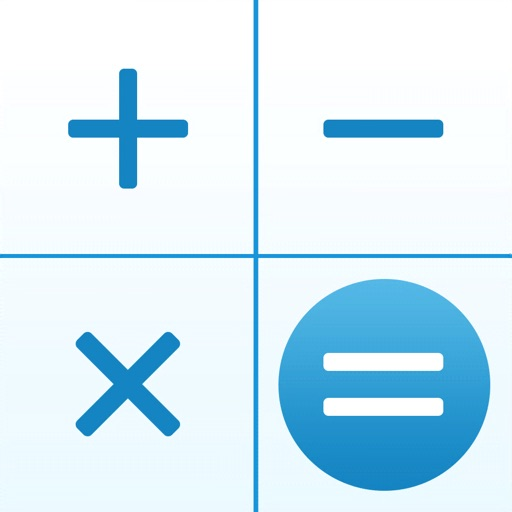## It is a calculator application which can calculate collectively by expressions like 100+30-50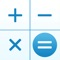# Calculator++P

by YUTAKA KENJO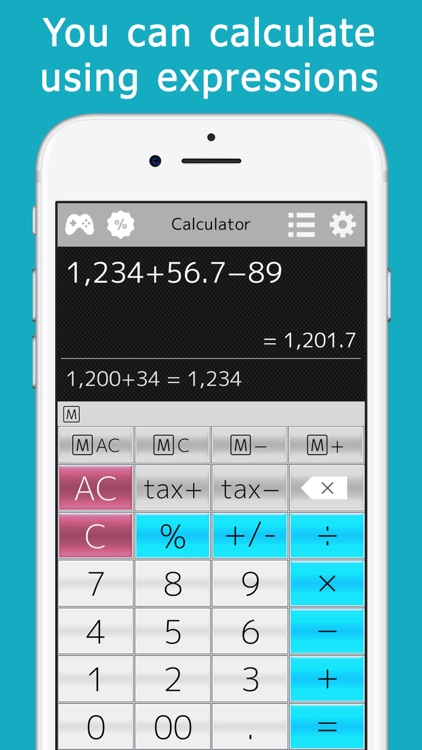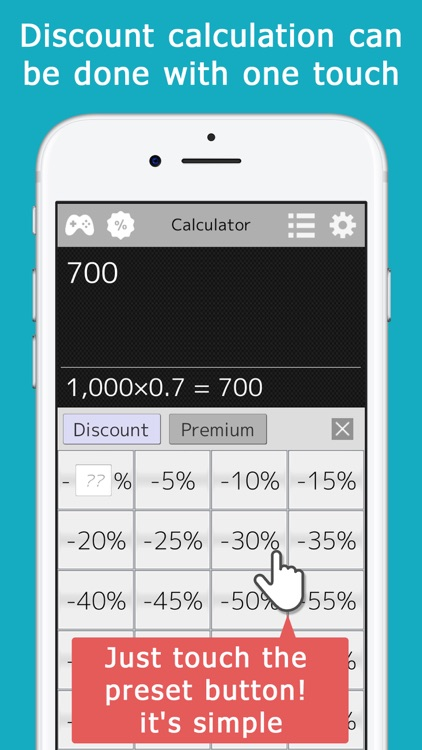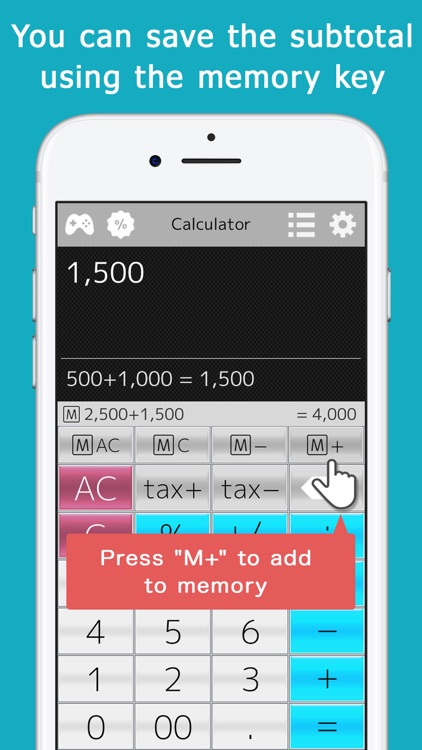It is a calculator application which can calculate collectively by expressions like 100+30-50.### App Details

Version
2.17
Rating
(4)
Size
41Mb
Genre
Utilities Finance
Last updated
April 29, 2022
Release date
January 12, 2016

### App Screenshots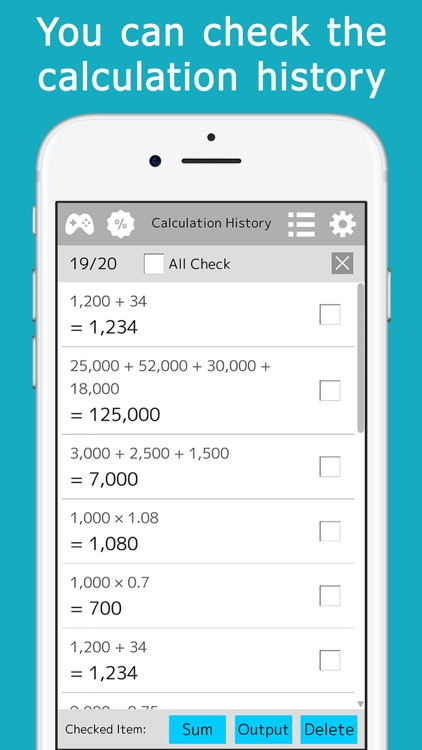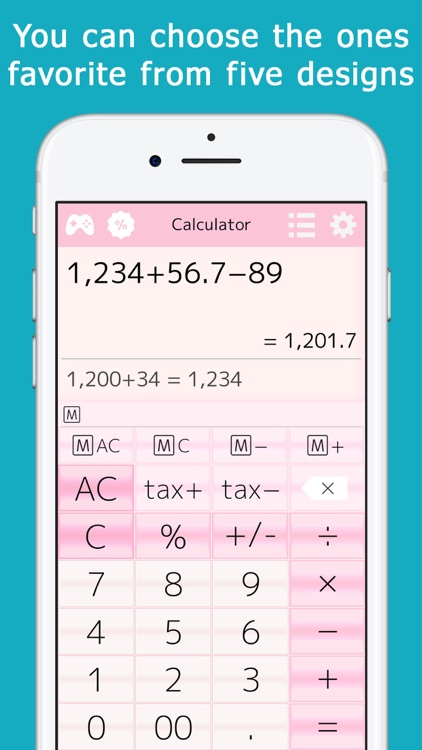### App Store Description

It is a calculator application which can calculate collectively by expressions like 100+30-50.
Discount / premium calculation can be done with one touch.
Calculation history is automatically recorded and can be confirmed at any time.

You can use it in various scenes of everyday such as shopping, tax calculation, estimate.
We emphasize easy-to-use and easy-to-view and it is simple.

By using the calculation history, it is released from the trouble of doing the same calculation and taking notes many times.
Also, you can reuse historical expressions and answers for calculation, so you can calculate efficiently.

On the history screen you can calculate the total of the answers.

Expressions are saved every time you enter them, so you can resume calculation immediately after you suspend or end the application.

The answers are displayed while you are entering the formula, so you can easily recalculate.

Discount calculation such as 20% and 30% discount can be done with one touch.
As buttons preset in units of 5%, such as 5%, 10%, 15% are prepared, you can easily calculate.
If you use the input box you can also calculate in 1% unit.

Moreover, it can calculate with VAT inclusion / VAT exclusion with one touch.
Multiple tax rates can be set.

If there are multiple tax rates, you can select the tax rate smoothly by touching the calculation button of the tax and then sliding it.

A memory key is installed.
This allows you to store a totalizer at each break of a calculation and calculate the total value.
It can be used conveniently for purchases, for example.

【Usage】
Press the "M +" key to add a sub-meter to the memory
Press the "M -" key to subtract the subtotal from the memory
Press the "M C" key to delete the last value stored in the memory (memory clear)
Press the "M AC" key to delete all the values stored in the memory (memory all clear)

If it is not use, you can turn it off from the setting.

A mini-game of calculation is carried and you can do a gymnastication of head in idle time etc.

[Function introduction]
· You can enter expressions and calculate them all together
· As with ordinary mathematical expressions, x and ÷ are calculated preferentially
· You can calculate using () (You need to make settings on the setting screen in order to display keys)
· As the expression becomes longer, the characters become smaller, so you can enter many numbers
· You can select the format of the decimal point and punctuation mark

12,345,678.9 (English-speaking countries, Japan, China, etc.)
12.345.678,9 (Germany, Italy, Spain, etc.)
12 345 678,9 (France, Russia, etc.)
1,23,45,678.9 (India etc.)

· Even if the application is interrupted or terminated during calculation, the formula at that time is saved, so you can resume calculation immediately
· By using the check box on the history screen, you can calculate the total value of answers
· Touch the history expression or answer, you can calculate by reusing those values
· "00" key is attached
· You can correct one character by backspace key
· VAT and discount · premium calculation can be done with one touch
· You can choose your favorite one from several designs
· You can set the key to right-justified or left-justified so that it can be operated with one hand even on a large screen
· You can select the number of decimal places to display
· It corresponds to the consumption tax reduction tax rate
· You can display it sideways on iPad